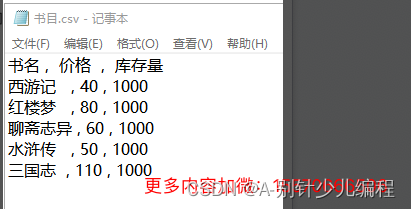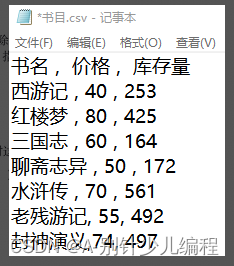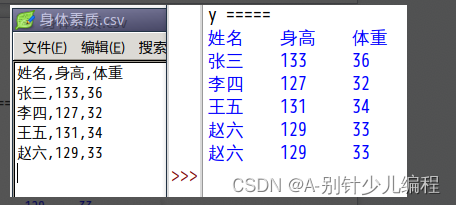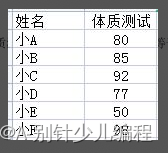## 一、单选题(共25题，共50分)

1.

c = [[‘赵大’,21,’男’,’北京’],[‘钱二’,20,’男’,’西安’],[‘孙三’,18,’女’,’南京’],[‘李四’,20,’女’,’杭州’]]
print(c)

 A. 男

 B. 北京

 C. 西安

 D. 女

2.f = open(“书目.csv” ,  )

print(a)

f.close

 A. “w”

 B. “a”

 C. “r”

 D. “a+”

3.with open(‘书目.csv’, ‘r’, encoding=’utf-8′) as f:

a = line.split(“,”)
if a == “水浒传” :
print(a)

 A. 老残游记

 B. 172

 C. 55

 D. 70

4.

a = [‘a’,’b’,’c’]
b = [1,2,3]
c = [b,a]
print(c)

 A. 1

 B. a

 C. ‘1’

 D. ‘a’

5.f = open(‘身体素质.csv’, ‘r’)

a = []

for i in f:

a.append(i.strip(‘\n’).split(‘,’))

f.close

for x in a:

line=”

for y in x:

line += ‘{ }    ‘.format(y)

print(line)

 A. \t

 B. \n

 C. \a

 D. \r

6.

 A. try

 B. except

 C. else

 D. finally

7.

try:

a=int(input())
b=int(input())
r=a/b
print(‘商是:’,r)
except:
print(‘错误’)
else:
print(‘正确’)
finally:
print(‘结束’)

16

8

 A. 错误

 B. 商是: 2.0 正确

 C. 商是: 2 正确 结束

 D. 商是: 2.0 正确 结束

8.

Python中将十进制20转化成八进制，我们可以使用下列哪个表达式？（　）

 A. bin(20)

 B. int(20)

 C. oct(20)

 D. hex(20)

9.

 A. 0x14转化为十进制,结果是20。

 B. 20转化成八进制，结果是0o20。

 C. 0x14转化为十进制,结果是22。

 D. 20转化成八进制，结果是0o30。

10.

 A. 十六进制转化为十进制，可以使用除16求余数，再顺序输出。

 B. 二进制转化为十进制，可以使用除2求余数，再逆序输出。

 C. 十六进制转化为十进制，可以使用除16求余数，再逆序输出。

 D. n位二进制能表示的最大十进制数是2的n次方减一。

11.

 A. bin(2022)

 B. int(2022)

 C. oct(2022)

 D. hex(2022)

12.

abs(-4.8)运行的结果是？（　）

 A. 4.8

 B. -4

 C. -5

 D. 5

13.

 A. ord( )函数的参数是整数。

 B. ord( )函数的参数是字符串类型。

 C. chr( )函数的参数是字符串类型。

 D. chr( )函数返回的数据类型是int。

14.

a=[34,7,8,23]

max(a)+abs(-5)

 A. 12

 B. 13

 C. 18

 D. 39

15.

set(‘小明你好’)运行的结果是？（　）

 A. [‘好’, ‘你’, ‘明’, ‘小’] # 列表中，文字顺序不固定

 B. (‘好’, ‘你’, ‘明’, ‘小’) #元组中，文字顺序不固定

 C. {‘好’, ‘你’, ‘明’, ‘小’} #集合中，文字顺序不固定

 D. {‘你’, ‘好’, ‘小’, ‘明’} #文字顺序固定

16.

a=[5,10,32,7]
for i in a:
print(i,”:”,bin(i))

 A. 分别输出5,10,32,7的二进制数。

 B. 分别输出5,10,32,7的八进制数。

 C. 分别输出5,10,32,7的在列表中的索引位置。

 D. 分别输出5,10,32,7的十六进制数。

17.

 A. len([1,2,3,4,5,789])

 B. max([78,6,5,67] )

 C. abs(-90)

 D. round(38.4)

18.

divmod(6,2)的运行结果是？（ ）

 A. 8

 B. 4

 C. （3,0）

 D. （0,3）

19.

 A. type（）

 B. dir()

 C. set()

 D. help()

20.

x=7

print(eval(“x+4”))

 A. 输出”x+4”

 B. 输出数字11

 C. 输出“7+4”

 D. 程序出错

21.

 A. 该函数是Python内置函数。

 B. 该函数可用于判断数据类型是否是数值类型。

 C. 该函数可将一个可遍历对象按照设置的序号组合成一个索引序列。

 D. 该函数通常用在for循环中，可返回一个内部由一个个元组组成的列表。

22.

enumerate()函数用于将一个可遍历的数据对象(如列表、元组或字符串)组合为一个索引序列。下列程序
example = ‘7890’
for i,j in enumerate(example):
print(i,j)

 A. 0,7 1,8 2,9 3,0

 B. （0 7） （1 8） （2 9） （3 0）

 C. 0 7 1 8 2 9 3 0

 D. （0,7） （1,8） （2,9） （3,0）

23.

 A. 顺序结构、选择结构、循环结构。

 B. if选择结构、for循环、while循环。

 C. 处理框、判断框、流程线。

 D. 自然语言、流程图、程序设计语言。

24.

“韩信点兵”是一个古老的数学问题，可以应用中国剩余定理进行求解。以下是使用计算机解决“韩信点兵”问题的几个步骤：
①编写Python程序，用计算机进行处理。
②设计“韩信点兵”求解算法。
③验证算法的功能和性能。
④分析问题，确定解题任务。

 A. ②④①③

 B. ④①②③

 C. ④②③①

 D. ④②①③

25.

 A. 枚举算法

 B. 解析算法

 C. 排序算法

 D. 对分查找算法

## 二、判断题(共10题，共20分)

26.

f = open(‘成绩.csv’, ‘r’)

for i in f:

a = i.strip(‘\n’).split(‘,’)

print(a)

f.close

f = open(‘成绩.csv’, ‘r’)

a = []

for i in f:

a.append(i.strip(‘\n’).split(‘,’))

f.close

print(a)

 正确 错误

27.

f = open(‘身体素质.csv’, ‘a’)

f.seek(0,0)  #用seek()方法，将文件指针移动到整个文件的最前面。

f.write(‘张三,133,36\n’)

f.close()

 正确 错误

28.

 正确 错误

29.

 正确 错误

30.

 正确 错误

31.

divmod（）函数执行结果是返回一个包含商和余数的元组。（　）

 正确 错误

32.

all（）函数用于判断给定的可迭代参数中的所有元素是否都为False,若是返回True,否则返回False。（　）

 正确 错误

33.

any()函数用于判断给定的可迭代参数中的所有元素是否都为True,若是返回True,否则返回False。（　）

 正确 错误

34.

 正确 错误

35.

list1 = [‘赵’,’钱’,’孙’,’李’,’周’,’吴’,’郑’,’王’]
print(list1[1:-4])
print(list1[1:4])

 正确 错误

## 三、编程题(共3题，共30分)

 36. 小明用冒泡排序算法编写了一段代码，请帮他把代码中红色标记的①②③处，补充完整。 a = [8,4,2,11,3,9] n = len(a) for i in range(0,     ①     ):     for j in range(0,     ②     ):         if a[j]>a[   ③   ]:             a[j],a[j+1] = a[j+1],a[j]       print(a) 试题编号：20220425-ll-037 试题类型：编程题 标准答案： 参考程序： a = [8,4,2,11,3,9] n = len(a) for i in range(0, n):     for j in range(0, n-i-1):         if a[j]>a[j+1]:             a[j],a[j+1] = a[j+1],a[j]       print(a) 试题难度：较难 试题解析： 评分标准： （1）n；   （2分） （2）n-i-1；（4分） （3）j+1 。 （4分）

 37. 下面程序，按字典序列统计出单词的个数，执行结果如下： are:2 first:2 happy:1 is:3 your:2 请补全下列代码中的①②③④四处。 a=[‘is’,’happy’,’is’,’your’,’are’,’first’,’first’,’is’,’are’,’your’] n=len(a)-1 for i in range(0,n):     for j in range(i+1,n+1):         if a[i]>a[j]:                   ①      key=a i=1 ans=1 while i<=n:     if key==a[i]:                ②             i+=1     else:         print(a[i-1]+’:’+str(ans))         key=a[i]                ③              i+=1        ④ 试题编号：20220504-ppz-023 试题类型：编程题 标准答案： 参考程序： a=[‘is’,’happy’,’is’,’your’,’are’,’first’,’first’,’is’,’are’,’your’] n=len(a)-1 for i in range(0,n):     for j in range(i+1,n+1):         if a[i]>a[j]:             a[i],a[j]=a[j],a[i] key=a i=1 ans=1 while i<=n:     if key==a[i]:         ans=ans+1         i+=1     else:         print(a[i-1]+’:’+str(ans))         key=a[i]         ans=1         i+=1print(a[i-1]+’:’+str(ans)) 试题难度：较难 试题解析： 评分标准： ①a[i],a[j]=a[j],a[i]；  （4分） ②ans=ans+1；（4分） ③ans=1；   （4分） ④print(a[i-1]+’:’+str(ans))。（4分）

 38. 请读取文件”cj.csv”的数据，数据内容如下图所示：下列代码实现了读取“姓名”和“体质测试”信息，输出“体质测试”考核为A的数量（大于等于85分）并显示相应同学姓名，请你补全代码。 import csv with open(‘cj.csv’) as f:      rows = list(  ①  )      n=0      for row in rows[1:]:         if   ②  :             print(row)             n+=1 print(n) 试题编号：20220512-xb-42 试题类型：编程题 标准答案： 参考程序： import csv with open(‘cj.csv’) as f:     rows = list(csv.reader(f))     n=0     for row in rows[1:]:         if int(row)>=85:             print(row)             n+=1 print(n) 试题难度：一般 试题解析： 评分标准： （1）csv.reader(f)； （2分） （2）int(row)>=85。（2分）